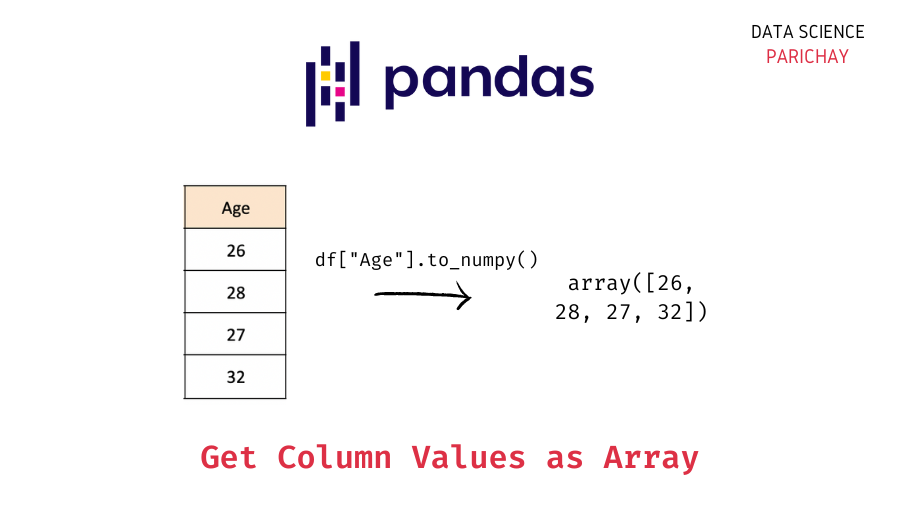# Pandas – Get Column Values as a Numpy Array

In this tutorial, we will know how to get the column values of a Pandas Dataframe as a Numpy Array.
Later, we will understand the same with the help of a few examples.

We can get the column values of a dataframe as a numpy array –

• by the `to_numpy()` method
• by the `to_records()` method

1. Using the `to_numpy()` method :

You can use the pandas series `to_numpy()` function to create a numpy array from the values of a pandas dataframe column.
We can directly apply the `to_numpy()` method to the column as shown in the syntax below.

Syntax: `dataFrameName['ColumnName'].to_numpy()`

2. Using the `to_records()` method.:

You can use the pandas dataframe `to_records()` method to first convert the dataframe to a numpy array (it returns a numpy `recarray` object, which you can think of as a numpy array that allows field access via attributes) and then access the specific column values as a 1d array.
The following is the syntax –
Syntax:

```  arrayname = dataFrameName.to_records()
arrayname["ColumnName"]````

Here as you can see, firstly we convert the entire dataframe into records, and then access the column that we want from that set of records (the two-dimensional numpy array).

📚 Data Science Programs By Skill Level

Introductory

Intermediate ⭐⭐⭐

🔎 Find Data Science Programs 👨‍💻 111,889 already enrolled

Disclaimer: Data Science Parichay is reader supported. When you purchase a course through a link on this site, we may earn a small commission at no additional cost to you. Earned commissions help support this website and its team of writers.

## Examples

We will now look at a few examples for a better understanding.

But before that, we will create a pandas dataframe that we will be using throughout this tutorial using the following command:

```import pandas as pd

# employee data
data = {
"Name": ["Jim", "Dwight", "Angela", "Tobi"],
"Age": [26, 28, 27, 32],
"Department": ["Sales", "Sales", "Accounting", "HR"]
}

# create pandas dataframe
df = pd.DataFrame(data)
# displays dataframe
df```

Output:

### Example 1: Using the `to_numpy()` function

Let’s get the values in the “Name” column as an array.

```# get column values as numpy array
df['Name'].to_numpy()```

Output:

`array(['Jim', 'Dwight', 'Angela', 'Tobi'], dtype=object)`

### Example 2: Using the `to_records()` function

```# convert dataframe to a recarray
arr = df.to_records()
# get column values as numpy array
arr['Name'] ```

Output:

`array(['Jim', 'Dwight', 'Angela', 'Tobi'], dtype=object)`

## Summary

In this tutorial, we looked at how to get column values as an array in a Pandas dataframe. The following are the methods covered –

• Use the pandas series `to_numpy()` method to get the values of a dataframe column as a numpy array.
• Alternatively, you can use the numpy `to_records()` function to create a numpy `recarray` object and then get the column values as a 1d array from it.

You might also be interested in –

•